Stata Tutorials & Resources:
Multiple Regression with Stata
Watch & Learn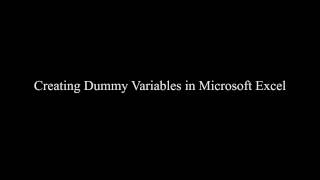How to Create Dummy Variables Taylor Smith demonstrates by converting categorical variable into dummy variables (7:25)Voting & Elections
Latin Am. Politics
Political Behavior
Teaching & Learning
International RelationsLinear Regression with Categorical Predictors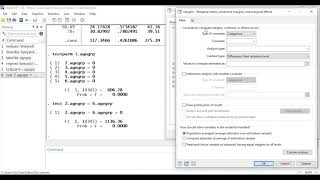From Stata Corp. (9:55)Multiple OLS Regression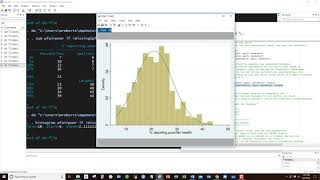In-depth tutorial, Wade Roberts (1:00:23)Linear Regression with Continuous Predictors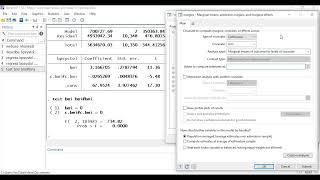From Stata Corp. (7:19)Pearsons r Correlation (listwise and pairwise deletion)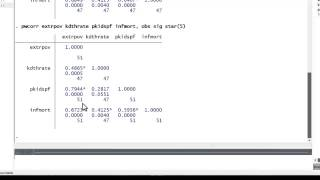Wade Roberts (7:09)Linear Regression with Categorical PredictorsFrom Stata Corp. (9:55)Multiple OLS RegressionIn-depth tutorial, Wade Roberts (1:00:23)